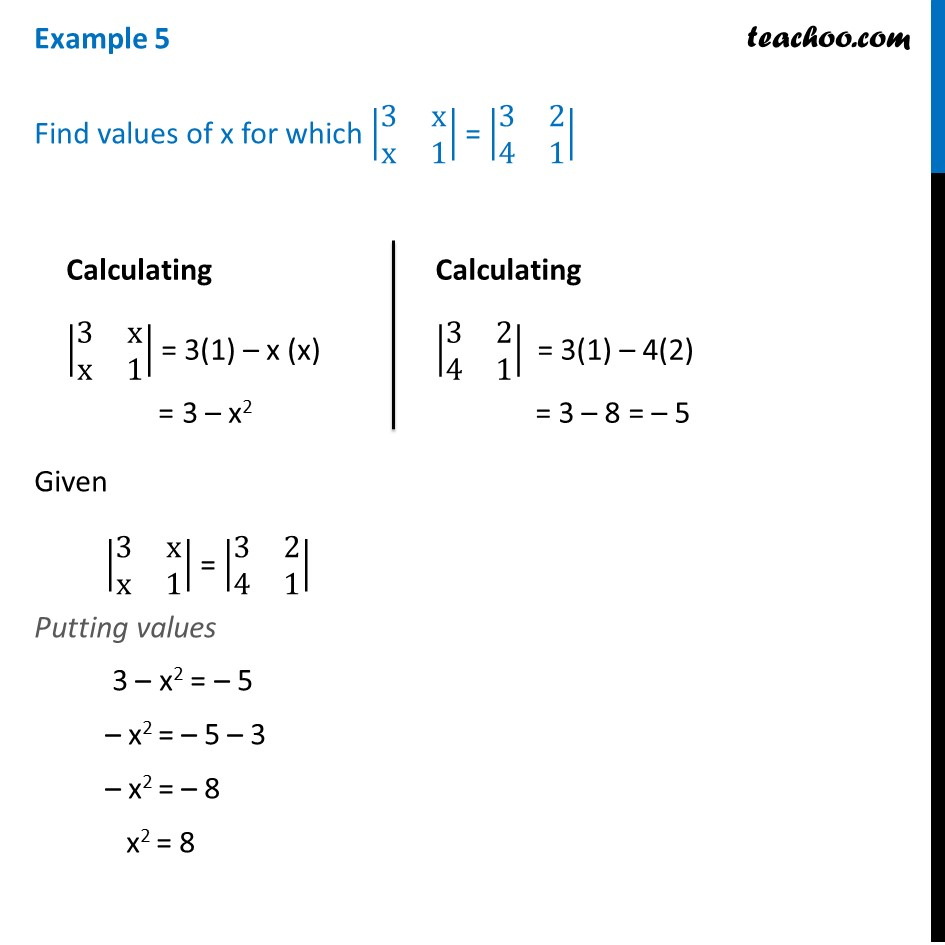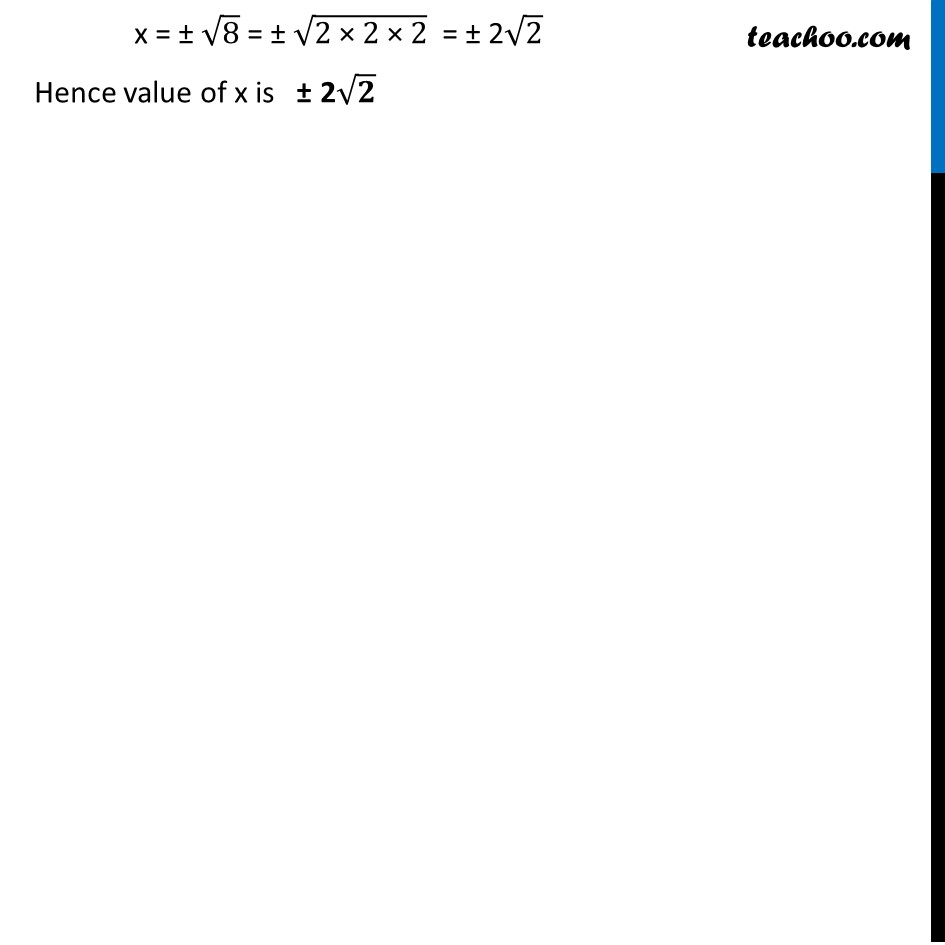Examples

Chapter 4 Class 12 Determinants
Serial order wiseLearn in your speed, with individual attention - Teachoo Maths 1-on-1 Class

### Transcript

Example 5 Find values of x for which |■8(3&x@x&1)| = |■8(3&2@4&1)| Given |■8(3&x@x&1)| = |■8(3&2@4&1)| Putting values 3 – x2 = – 5 – x2 = – 5 – 3 – x2 = – 8 x2 = 8 Calculating |■8(3&x@x&1)| = 3(1) – x (x) = 3 – x2 Calculating |■8(3&2@4&1)| = 3(1) – 4(2) = 3 – 8 = – 5 x = ± √8 = ± √(2" ×" 2" ×" 2) = ± 2√2 Hence value of x is ± 2√𝟐